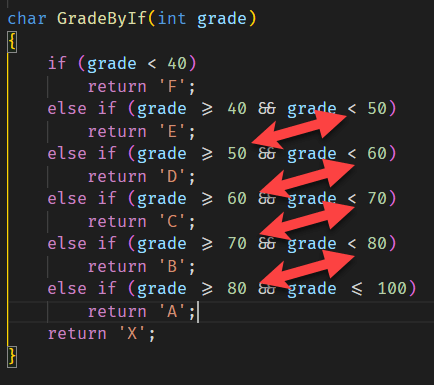Everyone who remembers their path towards becoming a programmer, either as a hobbyist or a career software engineer, will remember the problem of writing this program - given a mark, allocate a grade according to a table like this:

< 40 F
40 - 49 E
50 - 59 D
60 - 69 C
70 - 79 B
80 - 100 A
Other X

Your first attempt will probably look like this:

``````char GradeByIf(int grade)
{
if (grade < 40)
return 'F';
else if (grade >= 40 && grade < 50)
return 'E';
else if (grade >= 50 && grade < 60)
return 'D';
else if (grade >= 60 && grade < 70)
return 'C';
else if (grade >= 70 && grade < 80)
return 'B';
else if (grade >= 80 && grade <= 100)
return 'A';
return 'X';
}
``````

If you look at this closer you realize there is some repetition going on here:So the code can be simplified even further, if we remove the redundancy AND change the order of the conditions.

``````char GradeByIfV2(int grade)
{
if (grade > 100)
return 'X';
else if (grade >= 80)
return 'A';
else if (grade >= 70)
return 'B';
else if (grade >= 60)
return 'C';
else if (grade >= 50)
return 'D';
else if (grade >= 40)
return 'E';
else
return 'F';
}
``````

You can also use `switch` statements to achieve this goal. Like so:

``````char GradeBySwitchV1(int grade)
{
{
case int x when x < 40:
return 'F';
case int x when x >= 40 && x < 50:
return 'E';
case int x when x >= 50 && x < 60:
return 'D';
case int x when x >= 60 && x < 70:
return 'C';
case int x when x >= 70 && x < 80:
return 'B';
case int x when x >= 80 && x <= 100:
return 'A';
default:
return 'X';

}
}
``````

This can be simplified even further with a slight modification:

``````char GradeBySwitchV2(int grade)
{
{
case int x when x < 40:
return 'F';
case int x when x is >= 40 and < 50:
return 'E';
case int x when x is >= 50 and < 60:
return 'D';
case int x when x is >= 60 and < 70:
return 'C';
case int x when x is >= 70 and < 80:
return 'B';
case int x when x is >= 80 and <= 100:
return 'A';
default:
return 'X';

}
}
``````

Now these look almost identical:The `is` and `and` are not just syntactic sugar - they can also help the compiler find errors in your code.

So if in the IDE I introduce the following bug:

``````case int x when x is >= 40 and < 40:
return 'E';
``````

Note how the compiler notifies you after it attempts to evaluate your conditions:The compiler does not attempt to validate the previous version of the function.

C# 8 introduced switch expressions to simplify code like this still further:

``````char GradeBySwitchV3(int grade) =>
{
int x when x < 40 => 'F',
int x when x is >= 40 and < 50 => 'E',
int x when x is >= 50 and < 60 => 'D',
int x when x is >= 60 and < 70 => 'C',
int x when x is >= 70 and < 80 => 'B',
int x when x is >= 80 and <= 100 => 'A',
_ => 'X'
};
``````

Of interest is this part - `_ => 'X'`. Here `_ =>` is the equivalent of `default` in a traditional switch statement - it matches anything else.

As from the if example above, this can be simplified further:

``````char GradeBySwitchV4(int grade) =>
{
int x when x > 100 => 'X',
int x when x is >= 80 => 'A',
int x when x is >= 70 => 'B',
int x when x is >= 60 => 'C',
int x when x is >= 50 => 'D',
int x when x is >= 40 => 'E',
_ => 'F'
};
``````

It is still possible to simplify this code further, believe it or not! This is done by removing the variable declarations. This feature is available from C# 9 going forwards.

``````char GradeBySwitchV5(int grade) =>
{
< 40 => 'F',
>= 40 and < 50 => 'E',
>= 50 and < 60 => 'D',
>= 60 and < 70 => 'C',
>= 70 and < 80 => 'B',
>= 80 and <= 100 => 'A',
_ => 'X'
};
``````

And finally we can still simplify this to remove the redundancy to get our final version:

``````char GradeBySwitchV6(int grade) =>
{
> 100 => 'X',
>= 80 => 'A',
>= 70 => 'B',
>= 60 => 'C',
>= 50 => 'D',
>= 40 => 'E',
_ => 'F'
};
``````

If you are wondering at this terse, almost cryptic syntax - this is borrowed heavily from functional programming paradigm.

The equivalent program in F# would look like this:

``````let grade(x) = match x with
| x when x > 100 -> 'X'
| x when x >= 80 -> 'A'
| x when x >= 70 ->'B'
| x when x >= 60 ->'C'
| x when x >= 50 ->'D'
| x when x >= 40 ->'E'
| x when x < 40 ->'F'
| _-> 'X'
``````

The code is in my Github.

Happy hacking!